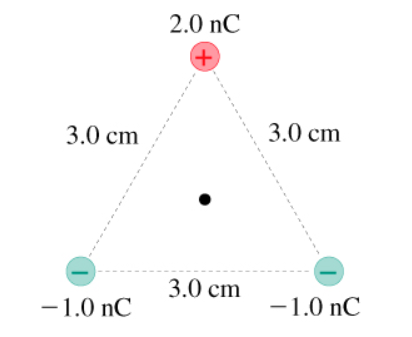# Problem: What is the electric potential at the point indicated with the dot in the figure? (Figure 1)Express your answer using two significant figures.

###### FREE Expert Solution

Electric potential:

$\overline{){\mathbf{V}}{\mathbf{=}}\frac{\mathbf{k}\mathbf{q}}{\mathbf{r}}}$

The distance r is given by properties of equilateral triangle:

r = a/sqrt(3)

r = (3.0)/sqrt(3) = 1.732 cm

97% (22 ratings)###### Problem DetailsWhat is the electric potential at the point indicated with the dot in the figure? (Figure 1)

Express your answer using two significant figures.

Frequently Asked Questions

What scientific concept do you need to know in order to solve this problem?

Our tutors have indicated that to solve this problem you will need to apply the Electric Potential concept. You can view video lessons to learn Electric Potential. Or if you need more Electric Potential practice, you can also practice Electric Potential practice problems.